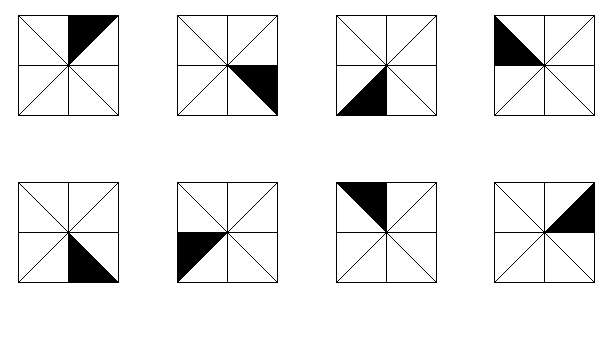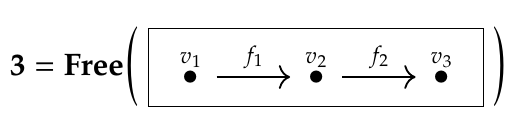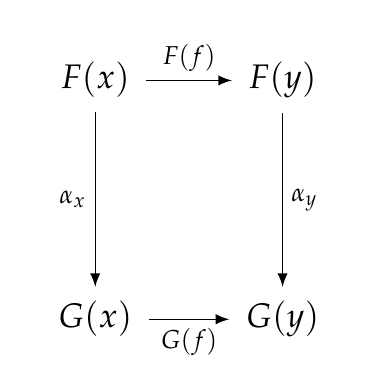Isomorphisms are very important in mathematics, and we can no longer put off talking about them. Intuitively, two objects are 'isomorphic' if they look the same. Category theory makes this precise and shifts the emphasis to the 'isomorphism' - the _way_ in which we match up these two objects, to see that they look the same. For example, any two of these squares look the same after you rotate and/or reflect them:An isomorphism between two of these squares is a _process of rotating and/or reflecting_ the first so it looks just like the second. As the name suggests, an isomorphism is a kind of morphism. Briefly, it's a morphism that you can 'undo'. It's a morphism that has an inverse: **Definition.** Given a morphism \$$f : x \to y\$$ in a category \$$\mathcal{C}\$$, an **inverse** of \$$f\$$ is a morphism \$$g: y \to x\$$ such that * \$$g\$$ is a **left inverse** of \$$f\$$: \$$g \circ f = 1_x \$$ and * \$$g\$$ is a **right inverse** of \$$f\$$: \$$f \circ g = 1_y \$$. I'm saying that \$$g\$$ is 'an' inverse of \$$f\$$ because in principle there could be more than one! But in fact, any morphism has at most one inverse, so we can talk about 'the' inverse of \$$f\$$ if it exists, and we call it \$$f^{-1}\$$. **Puzzle 140.** Prove that any morphism has at most one inverse. **Puzzle 141.** Give an example of a morphism in some category that has more than one left inverse. **Puzzle 142.** Give an example of a morphism in some category that has more than one right inverse. Now we're ready for isomorphisms! **Definition.** A morphism \$$f : x \to y\$$ is an **isomorphism** if it has an inverse. **Definition.** Two objects \$$x,y\$$ in a category \$$\mathcal{C}\$$ are **isomorphic** if there exists an isomorphism \$$f : x \to y\$$. Let's see some examples! The most important example for us now is a 'natural isomorphism', since we need those for our databases. But let's start off with something easier. Take your favorite categories and see what the isomorphisms in them are like! What's an isomorphism in the category \$$\mathbf{3}\$$? Remember, this is a free category on a graph:The morphisms in \$$\mathbf{3}\$$ are paths in this graph. We've got one path of length 2: $f_2 \circ f_1 : v_1 \to v_3$ two paths of length 1: $f_1 : v_1 \to v_2, \quad f_2 : v_2 \to v_3$ and - don't forget - three paths of length 0. These are the identity morphisms: $1_{v_1} : v_1 \to v_1, \quad 1_{v_2} : v_2 \to v_2, \quad 1_{v_3} : v_3 \to v_3.$ If you think about how composition works in this category you'll see that the only isomorphisms are the identity morphisms. Why? Because there's no way to compose two morphisms and get an identity morphism unless they're both that identity morphism! In intuitive terms, we can only move from left to right in this category, not backwards, so we can only 'undo' a morphism if it doesn't do anything at all - i.e., it's an identity morphism. We can generalize this observation. The key is that \$$\mathbf{3}\$$ is a poset. Remember, in our new way of thinking a **preorder** is a category where for any two objects \$$x\$$ and \$$y\$$ there is at most one morphism \$$f : x \to y\$$, in which case we can write \$$x \le y\$$. A **poset** is a preorder where if there's a morphism \$$f : x \to y\$$ and a morphism \$$g: x \to y\$$ then \$$x = y\$$. In other words, if \$$x \le y\$$ and \$$y \le x\$$ then \$$x = y\$$. **Puzzle 143.** Show that if a category \$$\mathcal{C}\$$ is a preorder, if there is a morphism \$$f : x \to y\$$ and a morphism \$$g: x \to y\$$ then \$$g\$$ is the inverse of \$$f\$$, so \$$x\$$ and \$$y\$$ are isomorphic. **Puzzle 144.** Show that if a category \$$\mathcal{C}\$$ is a poset, if there is a morphism \$$f : x \to y\$$ and a morphism \$$g: x \to y\$$ then both \$$f\$$ and \$$g\$$ are identity morphisms, so \$$x = y\$$. Puzzle 144 says that in a poset, the only isomorphisms are identities. Isomorphisms are a lot more interesting in the category \$$\mathbf{Set}\$$. Remember, this is the category where objects are sets and morphisms are functions. **Puzzle 145.** Show that every isomorphism in \$$\mathbf{Set}\$$ is a **bijection**, that is, a function that is one-to-one and onto. **Puzzle 146.** Show that every bijection is an isomorphism in \$$\mathbf{Set}\$$. So, in \$$\mathbf{Set}\$$ the isomorphisms are the bijections! So, there are lots of them. One more example: **Definition.** If \$$\mathcal{C}\$$ and \$$\mathcal{D}\$$ are categories, then an isomorphism in \$$\mathcal{D}^\mathcal{C}\$$ is called a **natural isomorphism.** This name makes sense! The objects in the so-called 'functor category' \$$\mathcal{D}^\mathcal{C}\$$ are functors from \$$\mathcal{C}\$$ to \$$\mathcal{D}\$$, and the morphisms between these are natural transformations. So, the _isomorphisms_ deserve to be called 'natural isomorphisms'. But what are they like? Given functors \$$F, G: \mathcal{C} \to \mathcal{D}\$$, a natural transformation \$$\alpha : F \to G\$$ is a choice of morphism $\alpha_x : F(x) \to G(x)$ for each object \$$x\$$ in \$$\mathcal{C}\$$, such that for each morphism \$$f : x \to y\$$ this naturality square commutes:Suppose \$$\alpha\$$ is an isomorphism. This says that it has an inverse \$$\beta: G \to F\$$. This \$$beta\$$ will be a choice of morphism $\beta_x : G(x) \to F(x)$ for each \$$x\$$, making a bunch of naturality squares commute. But saying that \$$\beta\$$ is the inverse of \$$\alpha\$$ means that $\beta \circ \alpha = 1_F \quad \textrm{ and } \alpha \circ \beta = 1_G .$ If you [remember how we compose natural transformations](https://forum.azimuthproject.org/discussion/2249/lecture-45-chapter-3-composing-natural-transformations/p1), you'll see this means $\beta_x \circ \alpha_x = 1_{F(x)} \quad \textrm{ and } \alpha_x \circ \beta_x = 1_{G(x)}$ for all \$$x\$$. So, for each \$$x\$$, \$$\beta_x\$$ is the inverse of \$$\alpha_x\$$. In short: if \$$\alpha\$$ is a natural isomorphism then \$$\alpha\$$ is a natural transformation such that \$$\alpha_x\$$ is an isomorphism for each \$$x\$$. But the converse is true, too! It takes a _little_ more work to prove, but not much. So, I'll leave it as a puzzle. **Puzzle 147.** Show that if \$$\alpha : F \Rightarrow G\$$ is a natural transformation such that \$$\alpha_x\$$ is an isomorphism for each \$$x\$$, then \$$\alpha\$$ is a natural isomorphism. Doing this will help you understand natural isomorphisms. But you also need examples! **Puzzle 148.** Create a category \$$\mathcal{C}\$$ as the free category on a graph. Give an example of two functors \$$F, G : \mathcal{C} \to \mathbf{Set}\$$ and a natural isomorphism \$$\alpha: F \Rightarrow G\$$. Think of \$$\mathcal{C}\$$ as a database schema, and \$$F,G\$$ as two databases built using this schema. In what way does the natural isomorphism between \$$F\$$ and \$$G\$$ make these databases 'the same'. They're not necessarily _equal!_ We should talk about this. **[To read other lectures go here.](http://www.azimuthproject.org/azimuth/show/Applied+Category+Theory#Chapter_3)**